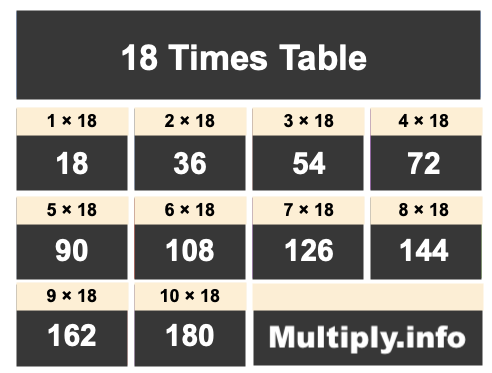18 Times TableThe picture above shows the first ten numbers in the 18 Times Table. It is created my multiplying 18 by one, then by two, then by three, and so on all the way up to ten.

Students and others use our 18 Times Table for many things, such as to find patterns or for memorizing products of numbers multiplied by 18.

In addition to the image of the 18 Times Table above, we have also written the 18 Times Table below showing 18 multiplied by every number up to one hundred. The list is in ascending order.

We created the 18 Times Table below to make it easy to read and print. We hope you will find our 18 Times Table helpful.

18 x  0 = 0
18 x  1 = 18
18 x  2 = 36
18 x  3 = 54
18 x  4 = 72
18 x  5 = 90
18 x  6 = 108
18 x  7 = 126
18 x  8 = 144
18 x  9 = 162
18 x 10 = 180
18 x 11 = 198
18 x 12 = 216
18 x 13 = 234
18 x 14 = 252
18 x 15 = 270
18 x 16 = 288
18 x 17 = 306
18 x 18 = 324
18 x 19 = 342
18 x 20 = 360
18 x 21 = 378
18 x 22 = 396
18 x 23 = 414
18 x 24 = 432
18 x 25 = 450
18 x 26 = 468
18 x 27 = 486
18 x 28 = 504
18 x 29 = 522
18 x 30 = 540
18 x 31 = 558
18 x 32 = 576
18 x 33 = 594
18 x 34 = 612
18 x 35 = 630
18 x 36 = 648
18 x 37 = 666
18 x 38 = 684
18 x 39 = 702
18 x 40 = 720
18 x 41 = 738
18 x 42 = 756
18 x 43 = 774
18 x 44 = 792
18 x 45 = 810
18 x 46 = 828
18 x 47 = 846
18 x 48 = 864
18 x 49 = 882
18 x 50 = 900
18 x 51 = 918
18 x 52 = 936
18 x 53 = 954
18 x 54 = 972
18 x 55 = 990
18 x 56 = 1008
18 x 57 = 1026
18 x 58 = 1044
18 x 59 = 1062
18 x 60 = 1080
18 x 61 = 1098
18 x 62 = 1116
18 x 63 = 1134
18 x 64 = 1152
18 x 65 = 1170
18 x 66 = 1188
18 x 67 = 1206
18 x 68 = 1224
18 x 69 = 1242
18 x 70 = 1260
18 x 71 = 1278
18 x 72 = 1296
18 x 73 = 1314
18 x 74 = 1332
18 x 75 = 1350
18 x 76 = 1368
18 x 77 = 1386
18 x 78 = 1404
18 x 79 = 1422
18 x 80 = 1440
18 x 81 = 1458
18 x 82 = 1476
18 x 83 = 1494
18 x 84 = 1512
18 x 85 = 1530
18 x 86 = 1548
18 x 87 = 1566
18 x 88 = 1584
18 x 89 = 1602
18 x 90 = 1620
18 x 91 = 1638
18 x 92 = 1656
18 x 93 = 1674
18 x 94 = 1692
18 x 95 = 1710
18 x 96 = 1728
18 x 97 = 1746
18 x 98 = 1764
18 x 99 = 1782
18 x 100 = 1800

Times Table Charts
Enter another number below to see the Times Table for that number.

19 Times Table
Did you find useful patterns in our 18 Times Table that you can use for the future? Check out the next Times Table on our list.

Copyright  |   Privacy Policy  |   Disclaimer  |   Contact